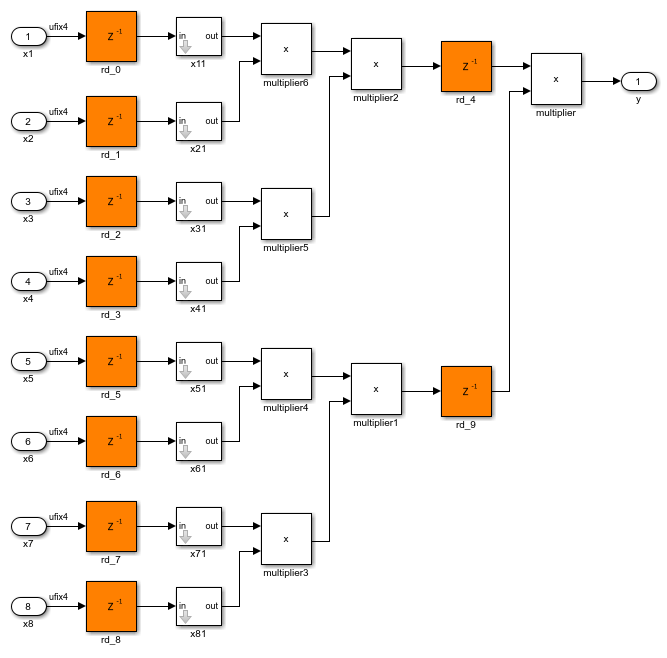# Use Distributed Pipelining Optimization in Models with MATLAB Function Blocks

This example shows how to optimize the generated HDL code for MATLAB Function blocks by using the distributed pipelining optimization. Distributed pipelining is an HDL Coder™ optimization that improves the generated HDL code from MATLAB Function blocks, Simulink® models, or Stateflow® charts. By using distributed pipelining, your design achieves higher clock rates on the FPGA device. For more information, see Distributed Pipelining.

### Open Multiplier Chain Model

This example shows how to distribute pipeline registers in a model that chains five multiplications.The `HDL_DUT` subsystem is the DUT for which you want to generate HDL code. The subsystem drives a MATLAB Function block `mult_chain`.To see the chain of multiplications, open the MATLAB Function block. The block contains this MATLAB code:

```function y = fcn(x1,x2,x3,x4,x5,x6,x7,x8) % A chained multiplication: % y = (x1*x2)*(x3*x4)*(x5*x6)*(x7*x8) ```
```y1 = x1 * x2; y2 = x3 * x4; y3 = x5 * x6; y4 = x7 * x8; ```
```y5 = y1 * y2; y6 = y3 * y4; ```
```y = y5 * y6; ```

### Apply Distributed Pipelining Optimization

1. Specify generation of two pipeline stages for the MATLAB Function block. Right-click the `mult_chain` MATLAB Function block. Select HDL Code > HDL Block Properties. In the HDL Properties window, set OutputPipeline to `2`.

2. Set Architecture to `MATLAB Datapath`. This architecture treats the MATLAB Function block like a regular subsystem. You can then apply various HDL Coder optimizations on MATLAB Function blocks along with other blocks in your Simulink model.

3. To enable the distributed pipelining optimization across hierarchies in your model, in the Configuration Parameters dialog box, click HDL Code Generation > Optimization. Click the Pipelining tab, then select the model configuration parameter Distributed pipelining.

4. To see the results of the optimization in the code generation report, click HDL Code Generation > Report. Then, enable the parameter Generate optimization report.

5. Optionally, when you use the MATLAB datapath architecture for a MATLAB Function block, you can enable synthesis timing estimates for distributed pipelining. Synthesis timing estimates calculate the propagation delays of the components in your design for distributed pipelining. Using this parameter, you can more accurately reflect how components function on hardware to better distribute pipelines in your design and maximize the clock frequency for your specific target device. For more information, see Distributed Pipelining Using Synthesis Timing Estimates.

To enable synthesis timing estimates, click HDL Code Generation > Optimization, then select the Pipelining tab. Enable the Use synthesis estimates for distributed pipelining parameter.

6. Generate HDL Code for the `HDL_DUT` subsystem. In the Apps tab, click HDL Coder. In the HDL Code tab, click Generate HDL Code.

By default, HDL Coder generates VHDL code in the `hdlsrc` folder.

### Analyze Results of Optimization

In the Code Generation Report window, in the Distributed Pipelining section, you see that the code generator moved the pipeline registers. To see the effects of the optimization, open the generated model `gm_hdlcoder_distpipe_multiplier_chain` and navigate to the `HDL_DUT` subsystem.The MATLAB datapath architecture replaces the MATLAB Function block with a subsystem. The optimization can then distribute the pipeline registers and the unit delay inside the subsystem to optimize the multiplier chain and improve timing. Open the `mult_chain` subsystem to view this optimization.